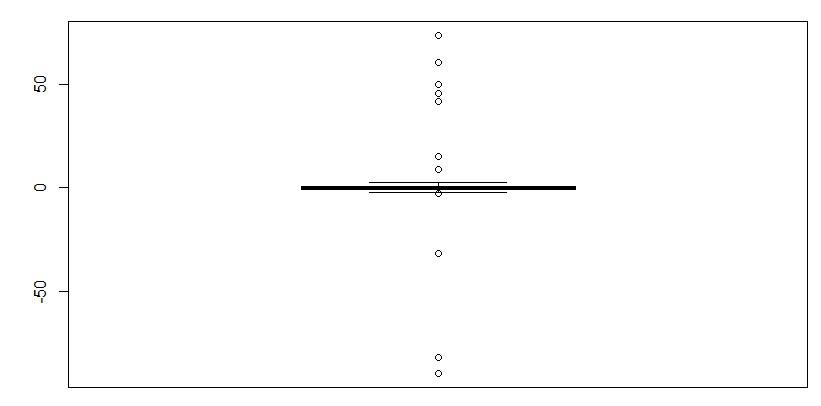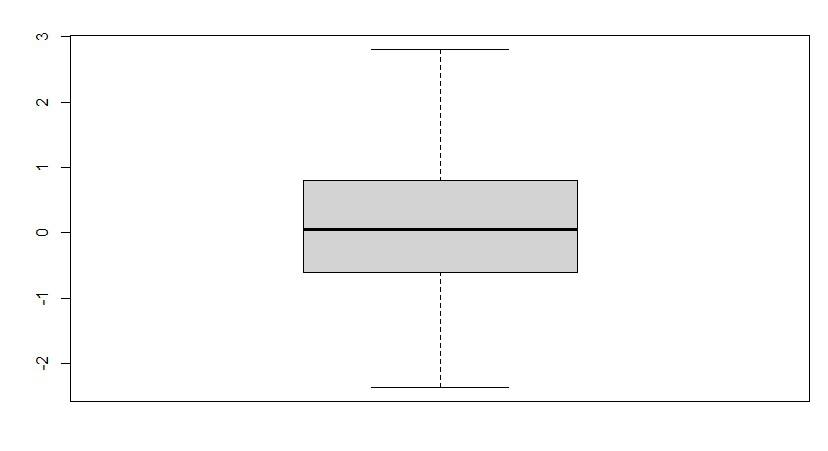Open in App
Not now

# Outlier Analysis in R

• Last Updated : 26 Oct, 2022

This article will focus on the outlier analysis, including Outlier detection and removal in the R programming language.

## What are outliers?

Data points far from the dataset’s other points are considered outliers. This refers to the data values dispersed among other data values and upsetting the dataset’s general distribution.

Effects of an outlier on model:

• The format of the data appears to be skewed.
• Modifies the mean, variance, and other statistical characteristics of the data’s overall distribution.
• Leads to the model’s accuracy level being biased.

## Steps involving Outlier Analysis:

Step 1: In this step, we will be, by default creating the data containing the outliner inside it using the rnorm() function and generating 500 different data points. Further, we will be adding 10 random outliers to this data.

## R

 `data <- ``rnorm``(500) ` `data[1:10] <- ``c``(46,9,15,-90, ` `         ``42,50,-82,74,61,-32)`

Step 2: In this step, we will be analyzing the outliner in the provided data using the boxplot, which will be plotting a barplot, and we will be able to analyze the outlier in the data. As said when reviewing a box plot, an outlier is defined as a data point that is located outside the whiskers of the box plot.

### boxplot() function:

Boxplots are created by using the boxplot() function in the R programming language.

Syntax: boxplot(x, data, notch, varwidth, names, main)

Parameters:

• x: This parameter sets as a vector or a formula.
• data: This parameter sets the data frame.
• notch: This parameter is the label for horizontal axis.
• varwidth: This parameter is a logical value. Set as true to draw width of the box proportionate to the sample size.
• main: This parameter is the title of the chart.
• names: This parameter are the group labels that will be showed under each boxplot.

## R

 `data <- ``rnorm``(500) ` `data[1:10] <- ``c``(46,9,15,-90, ` `         ``42,50,-82,74,61,-32)  ` `boxplot``(data)`

Output:Step 3: In this step, we will remove the outlier of the provided data boxplot.stats() function in R; the same illustration is shown in the below code.

## R

 `data <- data[!data %``in``% ``boxplot.stats``(data)\$out]`

Step 4: In this step, we will just verify if the outliner has been removed from the data simply by plotting the boxplot as done in step 2 and verifying it accordingly.

## R

 `data <- ``rnorm``(500) ` `data[1:10] <- ``c``(46,9,15,-90,42,50,-82,74,61,-32)  ` `data <- data[!data %``in``% ``boxplot.stats``(data)\$out] ` `boxplot``(data)`

Output:As we can see in the output plot that there is no outlier plotted in the plot. so, we successfully analyze and remove the outlier.

My Personal Notes arrow_drop_up
Related Articles